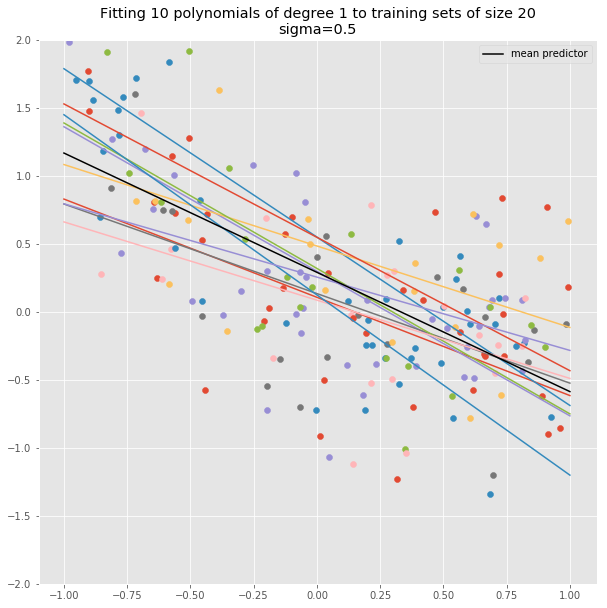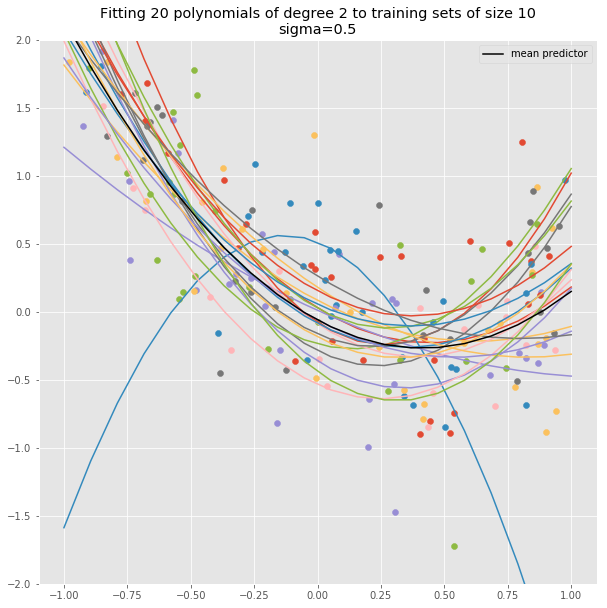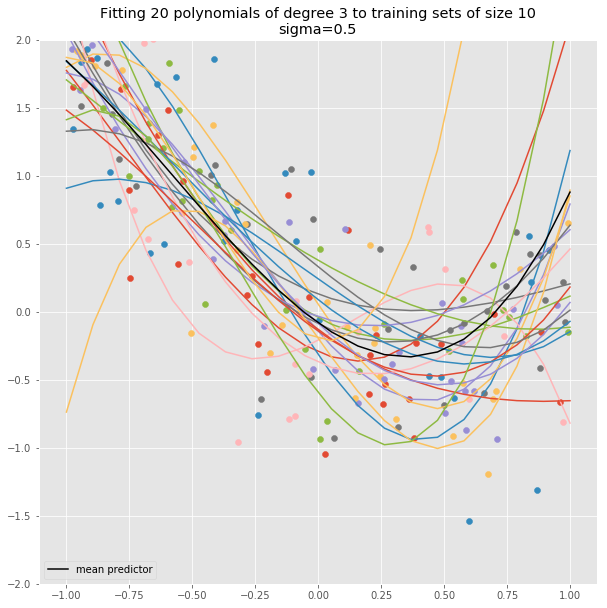## Bias and Variance¶

For the sake of this discussion, let's assume we are looking at a regression problem. For values of $x$ in the interval $[-1,1]$ there is a function $f(x)$ so that $$Y = f(x)+\epsilon$$ where $\epsilon$ is a noise term -- say, normally distributed with mean zero and variance $\sigma^2$.

We have a set $D$ of "training data" which is a collection of $N$ points $(x_i,y_i)$ drawn from the model and we want to try to reconstruct the function $f(x)$ so that we can accurately predict $f(x)$ for some new, test value of the function $x$.

Given $D$, we construct a function $h_{D}$ so as to minimize the mean squared error (or loss) $$L = \frac{1}{N}\sum_{i=1}^{N} (h_{D}(x_i)-y_i)^2.$$ This value is called the training loss or the training error.

Now suppose we pick a point $x_0$ and we'd like to understand the error in our prediction $h_{D}(x_0)$. We can ask what the expected value of the squared error $(h_D(x_0)-Y)^2$ where $Y$ is the random value obtained from the "true" model. First we write $$h_D(x_0)-Y = h_D(x_0)-E(Y)+E(Y)-Y$$ and use that $E(Y)=f(x_0)$ to get $$E((h_D(x_0)-Y)^2) = E((h_D(x_0)-f(x_0)^2)+E((E(Y)-Y)^2) + 2E((h_D(x_0)-E(Y))(E(Y)-Y)).$$ Since $E(Y)-Y=\epsilon$ is independent of $h_D(x_0)-E(Y)$ and $E(\epsilon)=0$ the third term vanishes and the second term is $\sigma^2$. We further split of the first term as $$(h_D(x_0)-f(x_0))=(h_D(x_0)-Eh_D(x_0)+Eh_D(x_0)-f(x_0)$$ where $Eh_D(x_0)$ is the average prediction at $x_0$ as $h$ varies over all possible training sets. From this we get $$E((h_D(x_0)-f(x_0))^2) = E((h_D(x_0)-Eh_D(x_0))^2) + E((f(x_0)-Eh_D(x_0))^2)$$ The first of these terms has nothing do do with the "true" function $f$; it measures how much the predicted value at $x_0$ varies as the training set varies. This term is called the variance. In the second term, the expectation is irrelevant because the term inside doesn't depend on the training set; it measures the (square of) the difference between the average predicted value and the value of $f(x_0)$; it is called the (squared) bias.

Putting all of this together, the error in prediction at a single point $x_0$ is made up of three terms:

• the underlying variance in the process that generated the data, $\sigma^2$.
• the sensitivity of the predictive function to the choice of training set (the variance)
• the degree to which the predictive function accurately guesses the true value on average.
In :
import numpy as np
from sklearn.preprocessing import PolynomialFeatures
from sklearn.linear_model import LinearRegression, Lasso
from sklearn.pipeline import make_pipeline
from numpy.random import normal,uniform
import matplotlib.pyplot as plt
plt.style.use('ggplot')

def sample(f, sigma=.5, N=5):
"""choose N x values as a training set from the interval at random and return f(x)+n(0,sigma^2) as the data at
that training set"""
x = uniform(-1,1,size=(N,1))
y = f(x)+normal(0,sigma,size=(N,1))
return np.concatenate([x,y],axis=1)

def bias_variance_plot(degree=2,samples=20,training_set_size=10,truth=None,sigma=.5):
plt.figure(figsize=(10,10))
if not truth:
truth = lambda x: 0
pipeline = make_pipeline(PolynomialFeatures(degree=degree),LinearRegression())
_=plt.title("Fitting {} polynomials of degree {} to training sets of size {}\nsigma={}".format(samples,degree,training_set_size,sigma))
x =np.linspace(-1,1,20)

plt.ylim([-2,2])
avg = np.zeros(x.shape)
for i in range(samples):
T= sample(truth,sigma=sigma,N=training_set_size)
plt.scatter(T[:,0],T[:,1])
model=pipeline.fit(T[:,0].reshape(-1,1),T[:,1])
y = model.predict(x.reshape(-1,1))
avg += y
_=plt.plot(x,model.predict(x.reshape(-1,1)))
_=plt.plot(x,avg/samples,color='black',label='mean predictor')
_=plt.legend()


Here are some examples. Suppose that the underlying data comes from the parabola $f(x)=-x+x^2$ with the standard deviation of the underlying error equal to $.5$.

First we underfit the data, by using a least squares fit of a linear equation, getting a group of fits that have high bias (the average fit doesn't match the data well) but the solutions don't vary much with the training set.

In :
bias_variance_plot(degree=1,training_set_size=20,samples=10,truth=lambda x: -x+x**2)Next we plot a quadratic fit, which has low bias (in fact the average fit is exactly right) and the variance is also controlled.

In :
bias_variance_plot(degree=2,training_set_size=10,samples=20,truth=lambda x: -x+x**2)Finally we overfit the training data using a degree 3 polynomial. Again, the bias is good -- in the limit the cubic fits average to the quadratic solution -- but now the variance is very high so there is a lot of dependence on the test set.

In :
bias_variance_plot(degree=3,training_set_size=10,samples=20,truth=lambda x: -x+x**2)In [ ]: